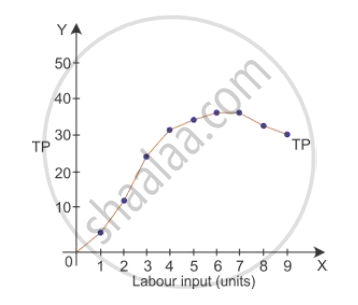# What Type of Production Function is this in Which Only One Input is Increased and Others Kept Constant? State the Behaviour of Total Product in this Production Function. - Economics

What type of production function is this in which only one input is increased and others kept constant? State the behaviour of total product in this production function.

#### Solution

Production function is the relationship between physical input such as labour, capital and physical output of a good. It is expressed in the following form: q = f (x1, x2). It means by using x1 amount of factor 1 and x2 amount of factor 2, you will be able to produce q amount of good.

Short run is a period of time during which some factors of production are fixed and some factors of production are variable. Production function in the short run is given as

q_x=f(x_1,barx_2)

where

q represents units of output produced

x1 represents capital units

barx_2" represent labour units"

Here, capital remains constant in the short run.

Behaviour of total product in the production function:-

Total Product (TP) is the total of each unit of the variable factor used in the process of production.

 Units of Variable Factor TP TP Curve 0 0 TP curve rises at an increasing rate 1 4 2 12 3 24 4 32 TP curve rises at an decreasing rate 5 34 6 36 TP curve remains constant 7 36 8 33 TP curve declines 9 30Let us consider the above schedule and the diagram. As more units of factor input, i.e. labour are used, TP tends to rise. Here, the total product increases at an increasing rate which is called increasing returns to the factor input till 3 units of factor input are used. However, when the 4th unit of factor input is used, the TP increases at a decreasing rate. TP remains constant from the 6th unit of factor input and TP starts declining from 36 to 33 when the 8th unit is employed.

Concept: Production Function - Short-run
Is there an error in this question or solution?
2015-2016 (March) Foreign Set 1
Share